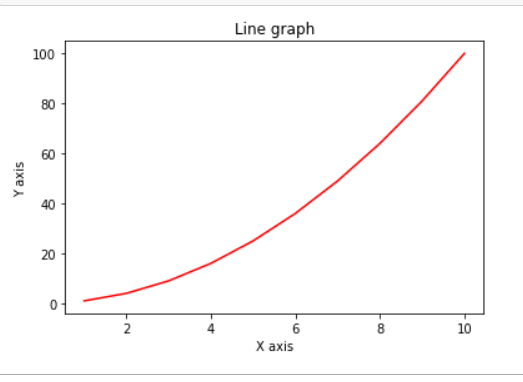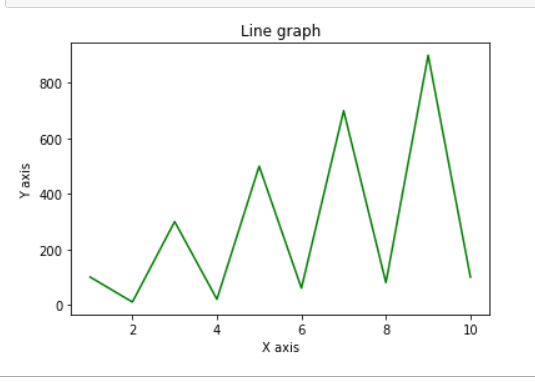# Plot line graph from NumPy array

• Difficulty Level : Easy
• Last Updated : 05 Aug, 2021

For plotting graphs in Python we will use the Matplotlib library. Matplotlib is used along with NumPy data to plot any type of graph. From matplotlib we use the specific function i.e. pyplot(), which is used to plot two-dimensional data.

Different functions used are explained below:

Attention geek! Strengthen your foundations with the Python Programming Foundation Course and learn the basics.

To begin with, your interview preparations Enhance your Data Structures concepts with the Python DS Course. And to begin with your Machine Learning Journey, join the Machine Learning - Basic Level Course

• np.arrange(start, end): This function returns equally spaced values from the interval [start, end).
• plt.title(): It is used to give title to the graph. Title is passed as the parameter to this function.
• plt.xlabel(): It sets the label name at X-axis. Name of X-axis is passed as argument to this function.
• plt.ylabel(): It sets the label name at Y-axis. Name of Y-axis is passed as argument to this function.
• plt.plot(): It plots the values of parameters passed to it together.
• plt.show(): It shows all the graph to the console.

Example 1 :

## Python3

 `# importing the modules``import` `numpy as np``import` `matplotlib.pyplot as plt` `# data to be plotted``x ``=` `np.arrange(``1``, ``11``)``y ``=` `x ``*` `x` `# plotting``plt.title(``"Line graph"``)``plt.xlabel(``"X axis"``)``plt.ylabel(``"Y axis"``)``plt.plot(x, y, color ``=``"red"``)``plt.show()`

Output :Example 2 :

## Python3

 `# importing the library``import` `numpy as np``import` `matplotlib.pyplot as plt` `# data to be plotted``x ``=` `np.arrange(``1``, ``11``)``y ``=` `np.array([``100``, ``10``, ``300``, ``20``, ``500``, ``60``, ``700``, ``80``, ``900``, ``100``])` `# plotting``plt.title(``"Line graph"``)``plt.xlabel(``"X axis"``)``plt.ylabel(``"Y axis"``)``plt.plot(x, y, color ``=``"green"``)``plt.show()`

Output :My Personal Notes arrow_drop_up